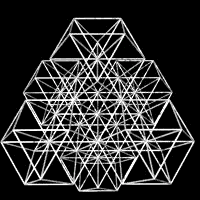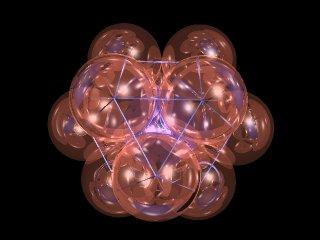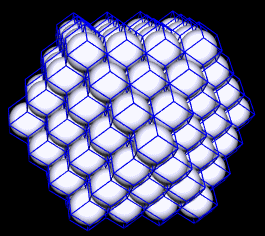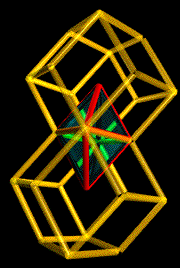The Isotropic Vector Matrix

Nature's Coordinate System

The tetrahedron combines with the octahedron to fill space, providing a lattice known to crystallographers as the face-centered cubic (fcc), and to Fuller as the isotropic vector matrix (IVM). Fuller emphasizes the IVM over the more familiar xyz apparatus, arguing that our fixation on cubes and right angles goes against the grain of nature, and begets a lot of unnecessary awkwardness in our curriculum.

This isotropic vector matrix is a lattice of rods connecting the centers of spheres of equal radius. Every sphere is a nucleus surrounded by 12 others in a cuboctahedral conformation (not the only option). The rods, or stress vectors, define tetrahedral and octahedral voids, of volume one and four respectively.

The IVM is also known as the octet truss and is quite widely used in architecture and engineering. As a uniform dispersion of points in space, the IVM models an ideal gas, freeze-framed to define average molecular positions as per Avogadro's Law.Twelve unit-radius IVM spheres closest pack around a nuclear sphere to form the twelve corners of the cuboctahedron of volume 20. When conceived as 24 circumferential pull-springs countering 24 radially paired push-springs, this arrangement of forces is also known as the vector equilibrium in synergetics.Additional layers of spheres, applied as shells of 42, 92, 162... 10 FF + 2 spheres, produce an ever larger agglomeration with the same cuboctahedral shape of radial depth F (where F = frequency).
 IVM spheres, if expanded uniformly towards one another to form planar interfaces with their neighbors, become space-filling rhombic dodecahedra, which have a volume of 6 relative to the unit-volume tetrahedron of prime vector edge length (prime vector = IVM sphere diameter). The corners of these rhombic dodecahedra occur in the voids between the spheres, at the ends of long and short face diagonals.Two adjacent sphere centers and the rhombic interface between them define an irregular space-filling octahedron of unit volume. This octahedron is named the Coupler in synergetics and may be assembled from 8 MITEs, or MInumum TEtrahedra (irregular, space-filling) of volume 1/8th. The voids between IVM spheres define the positions of three alternate IVMs, for a total of four. The octahedral voids, found at the ends of the rhombic dodecahedron's long face diagonals, define one alternate. The tetrahedral voids at the ends of the rhombic dodecahedron's short diagonals define two more. A smaller IVM, with edges 1/4th the original's, will pick up all these new sphere centers.Links: Most graphics by Richard Hawkins using Alias Animator V5.1 on an SGI Indigo2 Extreme; 3-frequency ping pong ball packing with rhombic dodecahedral casements by Kirby Urner using Visual FoxPro, and Povray Synergetics on the Web maintained by Kirby Urner